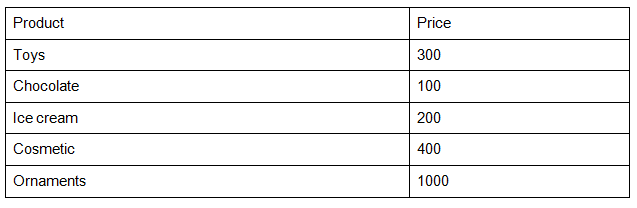# RRB ALP 2018 Practice Test Papers | Arithmetic Questions (Day-77)

Dear Aspirants, Here we have given the Important RRB ALP & Technicians Exam 2018 Practice Test Papers. Candidates those who are preparing for RRB ALP 2018 can practice these Arithmetic Questions to get more confidence to Crack RRB 2018 Examination.

[WpProQuiz 3091]

Click “Start Quiz” to attend these Questions and view Solutions

Direction(1 – 5): Read the following instructions and answer the question as follows In a Shop, Sita bought different kinds of things at certain rate as given table below1) What is the total amount spent by Sita?

a) 1500

b) 2000

c) 2500

d) 4000

2) What is the average amount spent for Ornaments and Cosmetics?

a) 200

b) 600

c) 700

d) 800

3) What is the ratio of amount spent for Chocolate and Icecreamto that of cosmetics?

a) 1: 1

b) 1: 2

c) 3: 4

d)  2: 1

4) What percentage of amount spent for toys compared to ornaments?

a) 30%

b) 10%

c) 20%

d) 25 %

5) If sita spent 40% of total amount she has how much amount left with her?

a) 1500

b) 3000

c) 4500

d) 6000

6) A man working 10 hours in 4 days he earns 1200. If he earns 1600 how many hours he want to work in 4 days?

a) 40/3 hours

b) 20/3 hours

c) 4 hours

d) 5.5 hours

7) Five year after ages of amit and vinit will be 30 and 35 respectively. What was the ratio of their ages after 10 years?

a) 8 : 7

b) 4 : 5

c) 7 : 8

d) 9 : 11

8) 8888 + 888 + 7777 + 777 = ?

a) 18900

b) 14500

c) 18330

d) 45000

9) 56% of 400 – 785 + 303 + 25^2= ?

a) 347

b) 367

c) 356

d) 345

10) x% of y = 200 % of x. Find the value of y

a) 100

b) 200

c) 300

d) 400

Total amount Spent by Sita = 300 + 100 + 200 + 400 + 1000 = 2000

Total amount spent for ornaments and Cosmetics = 1000 + 400 = 1400

Average = 1400/2 = 700

Chocolate + Ice cream : Cosmetics

100 +  200                    : 400

300 : 400

3:4

300*100/1000 = 30%

(2000*100)/60 = 3000

1200(x) = 1600 (10)

X = 40/3 hours

Five years after amit age = 30           Five years after Vinit age = 35

Present age of amit = 25                    Present age of Vinit        = 30

Age of 10 years after  = 35                 10 years after the age  = 40

Ratio

35 : 40

7 : 8

8888 + 888 + 7777 + 777 = 18330

56% of 400 – 785 + 303 + 25^2

= 224 – 785 + 303 + 625

= 1152 – 785 = 367

(x/y)*100 = 50x/100

100x/y = x/2

Y =  200

RRB ALP 2018 – “All in One” Study Materials and Practice Sets